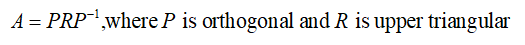# Suppose A = PRP–1, where P is orthogonal and R is upper triangular. Show that if A is symmetric, then R is symmetric and hence is actually a diagonal matrix.

Question
4 views

Suppose A = PRP–1, where P is orthogonal and R is upper triangular. Show that if A is symmetric, then R is symmetric and hence is actually a diagonal matrix.

check_circle

Step 1

According to the given information suppose,It is required to show that if A is symmetric then R is symmetric and hence is actually a diagonal matrix.

...

### Want to see the full answer?

See Solution

#### Want to see this answer and more?

Solutions are written by subject experts who are available 24/7. Questions are typically answered within 1 hour.*

See Solution
*Response times may vary by subject and question.
Tagged in